# A Language-Agent Approach To Formal Theorem-Proving

## 1. What is Formal Theorem Proving ?

Writing rigorous proofs has always been a challenging task that lies at the core of Mathematics. However, with theorems getting more and more complex, it takes months if not years to verify handwritten (informal) proofs. This challenge opens up a new window for automation by writing proofs and verifying proofs in a formal way. Besides mathematics, there are many more applications for writing formal proofs including the writing of certified programs in situations where we want a correctness guarantee for software.

However, writing proofs formally has been a daunting task given the amount of verbosity needed for it to be verifiable by a machine. It takes years’ worth of human work to write formal proofs. For example, it took multiple human years to finish the proofs for CompCert which is a verified compiler for C language. Despite all the difficulties involved, people have started moving towards writing formal proofs using proof assistants or Interactive Theorem Provers (ITPs). These are software tools designed to help humans write verbose proofs in a verifiable language. However, even with these assistants, it is too cumbersome to write complex proofs in formal languages. This makes it absolutely necessary to automate this task or at least provide greater assistance to humans who are writing such formal proofs.

There are multiple languages for theorem proving like Coq, Lean, Isabelle, ACL2, etc.


theorem mod_arith_2
(x : N) : x % 2 = 0 → (x * x) % 2 = 0 :=
begin
intro h,
rw nat.mul_mod,
rw h,
rw nat.zero_mul,
refl,
end


The figure above shows an example of proof written in Lean 3. This is a proof of the fact that for all natural numbers $x$, if $x$ is even then $x^2$ is also even.

(* Natural number definition. As per the definition, 3 = (S (S (S O)) *)
Inductive N : Set :=  O : N  (*Zero is a natural number*)
| S : N -> N. (*S is a function that returns the next natural number *)
(* addition over two natural numbers is defined recursively *)
Fixpoint add (n m : N) : N :=  match n with
| O => m  (* base case *)
| S n_minus_one => S (add n_minus_one m)  (* recursive case *)
end.
(* Adding a natural number to zero gives back the same natural number *)
Theorem add_n_O : forall n : N, add n O = n.
Proof.
(*[Goal]<forall n : N, add n O = n>*)
intros n.

induction n.

simpl.
(*[NextGoal]<O = O>*)

(*[Goal]<O = O>*)
reflexivity.
(*[NextGoal]<add (S n) O = S n>*)

(*[Goal]<add (S n) O = S n>*)
simpl.
(*[NextGoal]<S (add n O) = S n>*)

(*[Goal]<S (add n O) = S n>*)
rewrite -> IHn.
(*[NextGoal] <S n = S n> *)

(*[Goal]<S n = S n> *)
reflexivity.
(*[NextGoal]<Proof finished>*)
Qed.


The figure above shows a formal proof written in Coq language, and how the proof goal changes with application of each proof-tactic. This also shows the formalization of natural numbers and a simple proof of the fact that for all natural numbers $n$, $n + 0 = n$.

## 2. Automatic Theorem Proving History

### 2.1 Proof Theory: Brief History

The proof theory tries to represent proofs as formal mathematical objects which can then be analyzed using mathematical tools. The formalization of proofs was first attempted by David Hilbert and Paul Bernays in their book called “Grundlagen der Mathematik” in 1934-36. However, the mechanization of proofs was not successfully attempted until the 1970s when Nqthm was created by Boyer and Moore. Nqthm was the precursor to ACL2. ACL2 has been widely used in the industry, for example, by AMD to verify the correctness of floating-point operations. Later in the twenty-first century, more sophisticated proof languages like Coq and Lean were developed.

### 2.1 Automation and ITPs

Since we can express proofs in a formal language, it seems natural to describe the problem of proving as a search problem. The search space is the set of all possible proofs which can be written in this formal language. However, the search space is too large to be explored by a human. This is where automation comes into the picture. Automation can be used to explore the search space and find proofs. This is the idea behind Automated Theorem Proving (ATP).

### 2.2 ATP and Machine Learning:

Given the vast space of possible proofs in formal language. It seems reasonable to use ML to narrow down the search space for finding proofs. We can categorize the work done in this field into the following categories:

• Guided Proof Search
• Train for Next Proof-Step Prediction + Search for sequence of proof-steps: These approaches use supervised learning for predicting the next-proof step and then use this trained model to guide a search technique, e.g., best-first or depth-limited search, that synthesizes a proof. These methods were first based off on small slace neural networks (Yang & Deng, 2019; Sanchez-Stern et al., 2020; Huang et al., 2019) as predictors. More recent methods, such as GPT-f (Polu & Sutskever, 2020), PACT (Han et al., 2021), HyperTree Proof Search (Lample et al., 2022), and REPROVER (Yang et al., 2023), have used LLMs.
• Proof prediction using informal proofs: The approach Draft-Sketch-Proof (Jiang et al., 2022) method relies on informal proofs to generate formal proofs.
• Proof prediction using error signals: Methods like Baldur (First et al., 2023) generate the whole proof in one shot using an LLM and then repair it.
• End-to-End Proof Search: These methods use reinforcement learning to directly search for proofs. Kaliszyk et al. (2018) pioneered the use of RL in theorem-proving; subsequently, Wu et al. (2021) gave TacticZero, a deep RL approach to the problem.

### 2.3. Use of LLMs as Language Agents

Several distinct LLM agent architectures have been proposed over the last year (AutoGPT; ReAct by Yao et al., 2022; Reflection by Shinn et al., 2023; Voyager by Wang et al., 2023). These models combine an LLM’s capability to use tools (Toolformer by Schick et al. (2023)), decompose tasks into subtasks, and self-reflect.

## 4. CoPrA: in-COntext PRover Agent

We introduce a new approach in-Context Prover Agent (COPRA) which is a Language Agent for ATP.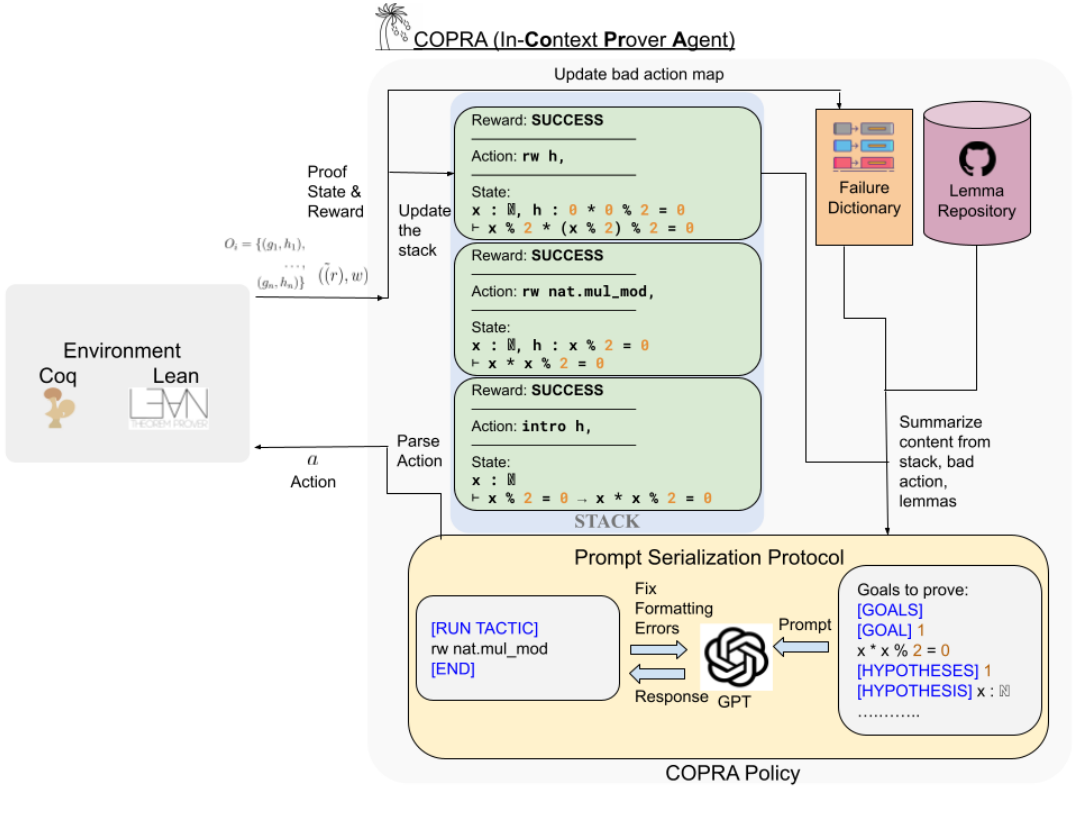The image above shows how our approach works. We propose a novel theorem proving agent which uses history of previous proof states, failures, repository of useful lemmas, and LLM as part of a verbal policy to predict the next proof action which will lead to simplification of the proof-state. Full details of our approach is described in our paper A Language-Agent Approach To Formal Theorem-Proving (CoPrA).

(On a side-note: Copra actually means the dried, white flesh of the coconut from which coconut oil is extracted)

### 4.1 Approach

Our approach is based on the following ideas:

1. Use a stack to store the history of proof states and proof actions.
2. Have a map for storing a map of proof states to bad proof actions.
3. A Prompt Serialization Protocol (PSP) helps in laying out the history of proof actions, proof states, and textual rewards.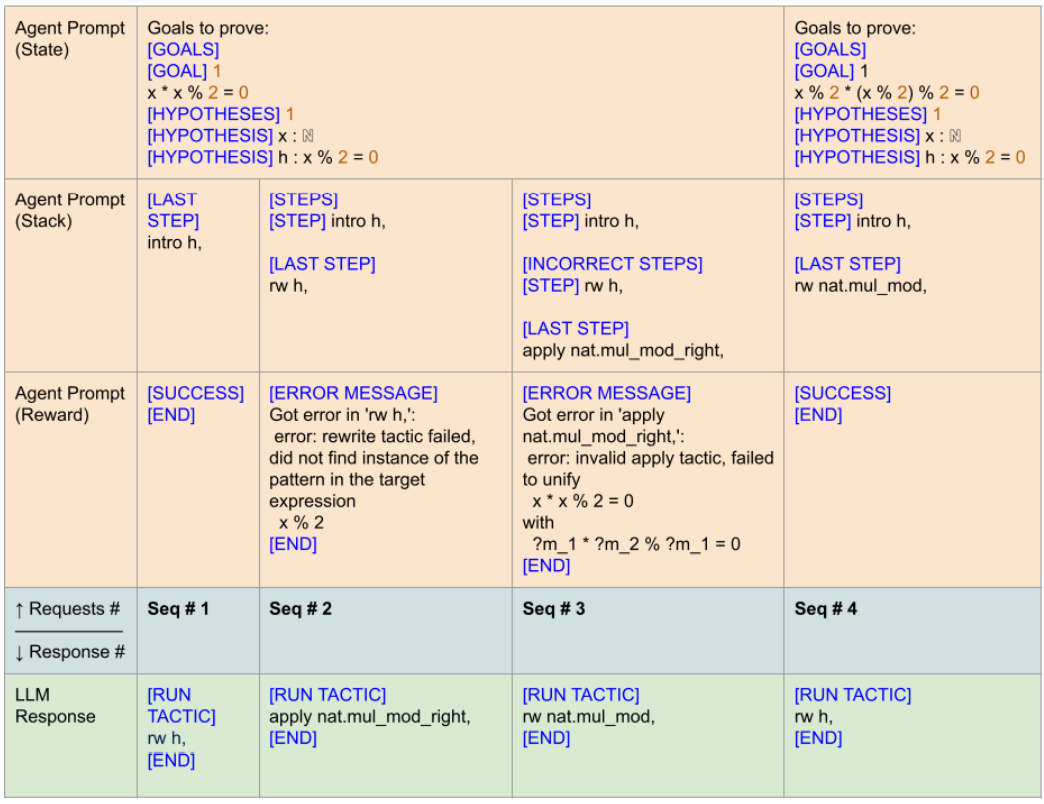In the above figure, Seq #1-#4 represent distinct invocations of the LLM. In each invocation, PSP first generates the “agent prompt,” which consists of three parts. The first part (“state”) is simply a serialization of the current proof state. The second (“stack”) incorporates information about previous actions as well as the bad actions for the current proof state. The third (“reward”) encodes the feedback from the environment regarding the success or failure of the last action. The response of the LLM to this prompt is then translated into a proof action. This action is then executed on the theorem prover.

### 4.2 Evaluation

We evaluated our approach on two datasets: miniF2F and CompCert which belong to different domains mathematics and software verification respectively.

We use a metric called “Pass@k-inference” while comparing our approach with ReProver and Proverbot9001. The standard metric for evaluating theorem-provers is pass@k. In this metric, a prover is given a budget of k proof attempts; the method is considered successful if one of these attempts leads to success. However, a key objective of our research is to discover proofs quickly, with fewer LLM queries and lower wall-clock time. The pass@k metric does not evaluate this characteristic as it does not quantify the number of LLM queries or amount of time needed by a proof attempt.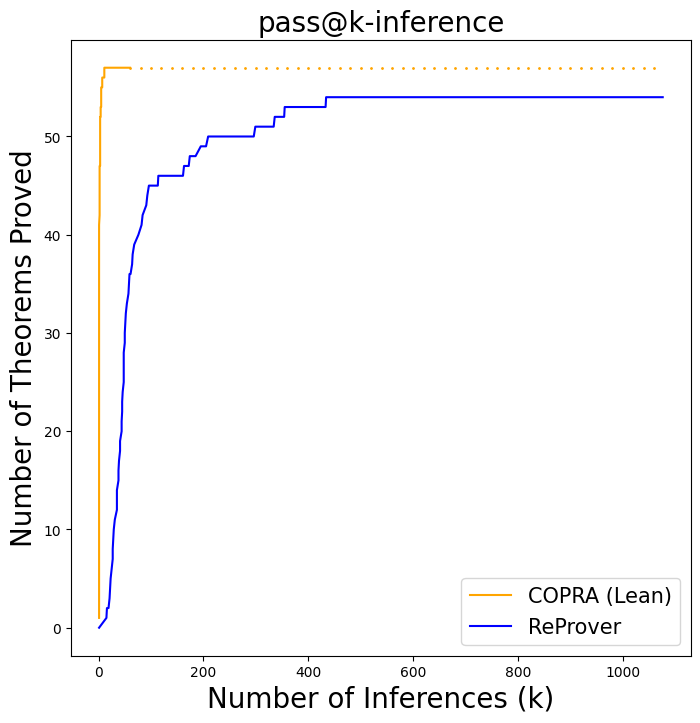The figure shows, comparison between ReProver and our approach on miniF2F dataset. It shows the number of proofs solved by CoPrA and ReProver as a function of the number of inference steps. CoPrA proves a lot more theorems in just 60 inference steps.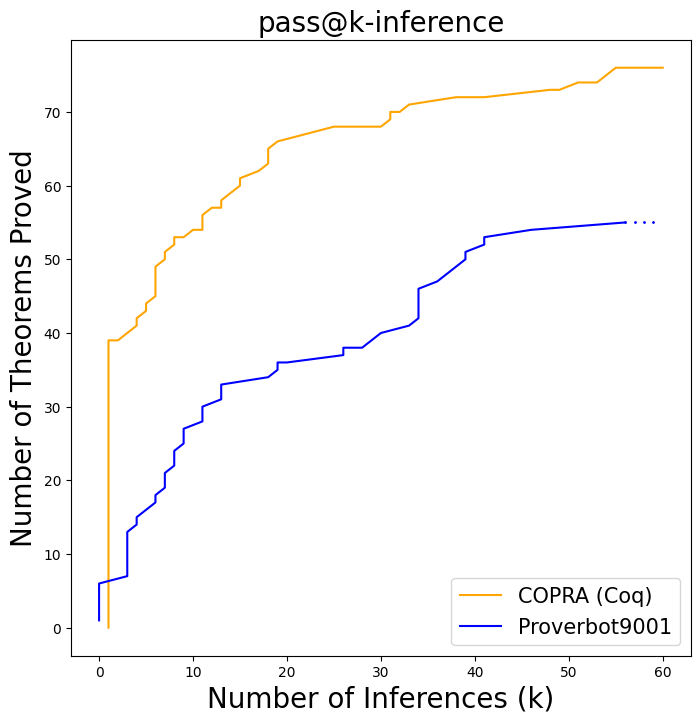The figure shows, comparison between Proverbot9001 and our approach on CompCert dataset. It shows the number of proofs solved by CoPrA and Proverbot9001 as a function of the number of inference steps. CoPrA proves a lot more theorems in just 60 inference steps.

The tables below shows a detailed comparison of our approach with ReProver and Proverbot9001.From Table 3, we can see that backtracking is important for proving longer theorems. From these tables, it is clear that our approach is not able to find the proofs fast, but also give up proving much faster when the theorem is hard enough.

### 4.3 Some interesting proofs found by CoPrAThe above image shows, some proofs in Lean found by CoPrA on miniF2F dataset.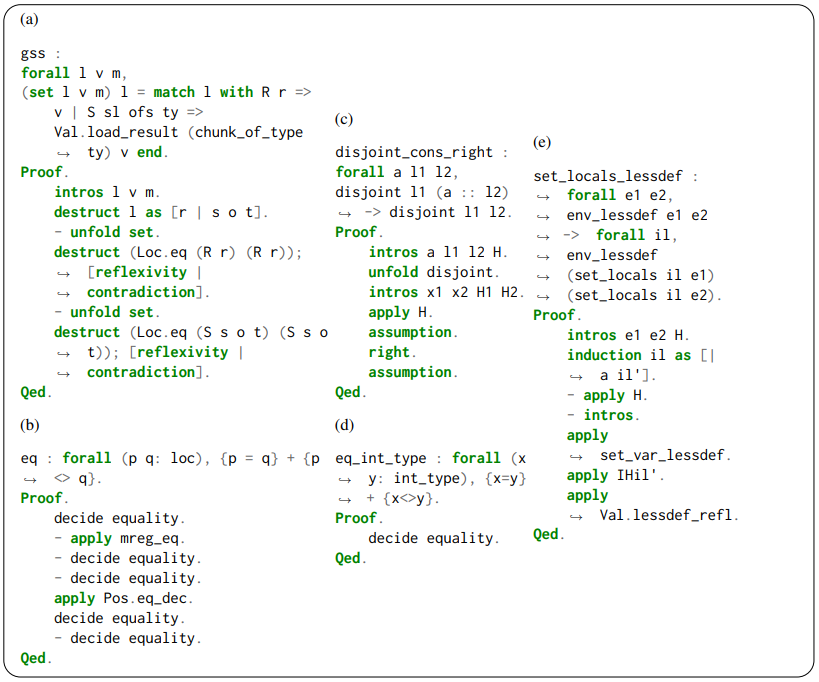The above image shows, some proofs in Coq found by CoPrA on CompCert dataset.

Share: